Q-Chem 5.0 User’s Manual

# 10.6 Electrostatic Potentials

Q-Chem can evaluate electrostatic potentials on a grid of points. Electrostatic potential evaluation is controlled by the \$rem variable IGDESP, as documented below.

IGDESP
 Controls evaluation of the electrostatic potential on a grid of points. If enabled, the output is in an ASCII file, plot.esp, in the format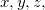esp for each point.

TYPE:
 INTEGER

DEFAULT:
 none no electrostatic potential evaluation

OPTIONS: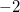same as the option ’-1’, plus evaluate the ESP of \$external_charges\$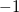read grid input via the \$plots section of the input deck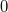Generate the ESP values at all nuclear positions +readgrid points in bohr from the ASCII file ESPGrid

RECOMMENDATION:
 None

The following example illustrates the evaluation of electrostatic potentials on a grid, note that IANLTY must also be set to 200.

Example 10.245  A job that evaluates the electrostatic potential for Hon a 1 by 1 by 15 grid, along the bond axis. The output is in an ASCII file called plot.esp, which lists for each grid point,,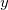,, and the electrostatic potential.

```\$molecule
0  1
H   0.0   0.0   0.35
H   0.0   0.0  -0.35
\$end

\$rem
METHOD     hf
BASIS      6-31g**
IANLTY     200
IGDESP     -1
\$end

\$plots
plot the HOMO and the LUMO on a line
1   0.0   0.0
1   0.0   0.0
15  -3.0   3.0
0  0  0  0
0
\$end
```

We can also compute the electrostatic potential for the transition density, which can be used, for example, to compute the Coulomb coupling in excitation energy transfer.

ESP_TRANS
 Controls the calculation of the electrostatic potential of the transition density

TYPE:
 LOGICAL

DEFAULT:
 FALSE

OPTIONS:
 TRUE compute the electrostatic potential of the excited state transition density FALSE compute the electrostatic potential of the excited state electronic density

RECOMMENDATION:
 NONE

The electrostatic potential is a complicated object and it is not uncommon to model it using a simplified representation based on atomic charges. For this purpose it is well known that Mulliken charges perform very poorly. Several definitions of ESP-derived atomic charges have been given in the literature, however, most of them rely on a least-squares fitting of the ESP evaluated on a selection of grid points. Although these grid points are usually chosen so that the ESP is well modeled in the “chemically important” region, it still remains that the calculated charges will change if the molecule is rotated. Recently an efficient rotationally invariant algorithm was proposed  that sought to model the ESP not by direct fitting, but by fitting to the multipole moments. By doing so it was found that the fit to the ESP was superior to methods that relied on direct fitting of the ESP. The calculation requires the traceless form of the multipole moments and these are also printed out during the course of the calculations. To request these multipole-derived charges the following \$rem option should be set:

MM_CHARGES
 Requests the calculation of multipole-derived charges (MDCs).

TYPE:
 LOGICAL

DEFAULT:
 FALSE

OPTIONS:
 TRUE Calculates the MDCs and also the traceless form of the multipole moments

RECOMMENDATION:
 Set to TRUE if MDCs or the traceless form of the multipole moments are desired. The calculation does not take long.